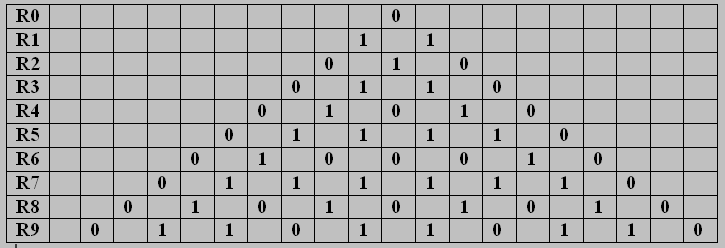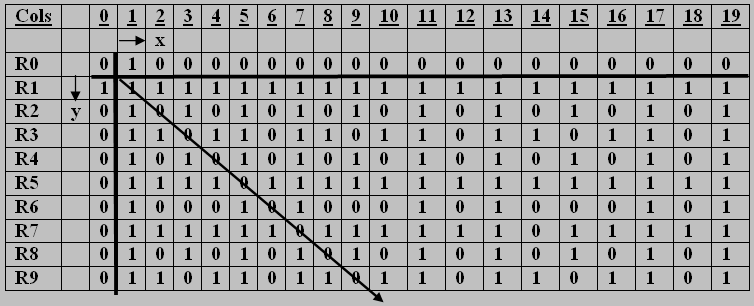## CNB4 The Cycle-Number Matrix C

August 17th, 2012

Morning!

Now that you are familiar with the cycle-number triangle T, I will show you a matrix which I call C*, which is obtained simply by turning T on its side. You must be familiar with this operation, as it is often done with Pascal’s triangle. It is sometimes (often?) more convenient to study the elements by having them in standard matrix form. To picture C*, you can think of T with a right-angle at its apex, being rotated anti-clockwise through 45 degrees.

Once we have done this, we can imagine our matrix as being an array of elements situated in the Euclidean plane, and refer them to a Cartesian reference frame. This will have Origin at cycle-number zero, and the positive x-axis horizontal as usual through O, whilst the positive y-axis  goes through O but points downwards.  Below are two diagrams, the upper one being T(9), and the lower one being C*. I call C* the augmented C-matrix; it is turned into C by removing  column C0 and row R0. To refer to C‘s elements, move the reference frame so that the origin is in cell (1,1), with no change to the axes except that now x = 1,2,3, … and y = 1,2,3, …

Note that I continue to show the grid lines, but for convenience of reference only. The shown elements (all from {0,1}) are to be thought of as being points in the centres of the grid cells.

Table 1 :  The circle-number triangle T(9)You have already done some looking at and studying (0,1)-patterns in triangle T.  So please turn now to the same kinds of study of patterns occurring in matrix C. I will ask a few questions below the table.

Table 2:  The cycle-number matrix C(9, infinity),  seen as a submatrix of C*Now you can see the start of my cycle-number sequence, with 1 appearing in row R1 , 2 appearing in row R2, and so on. Of course, you can only see bits of them in Table 2, as far as the matrix will show you. By the way, I use a special name for a submatrix of C which starts at the upper left corner of C and has r rows and s columns: I call such submatrices Blocks; so what you can see is Block B(r,s) = B(9, 19). Note the diagonal line, which is the leading diagonal (l.d.) of natrix C.

Now let me ask those promised questions. We can find and study patterns in lines, triangles, squares and so on. As you will learn if you do questions 1 to 5 below.

(q1) What patterns do you find in lines y = 3 , y=6, y=5, y=12, y=13, y=18 ?

(q2) What patterns do you find in lines y=x, y=x+1, y=x+2, y=x+3? How about y=x-1, y=x-2, …?

(q3) Can you find a triangle each of whose sides has pattern 111 ? can you find two such?

(q4) Can you find more than one 111 triangle? Can you find (and prove!)an infinity of them?

(q5) Let us call these equi-side-patterned triangles (espt’s, say). Can you find espt’s with side-patterns 1111, 11111, 111111, … etc. Can you suggest (and prove!) general theorems about this kind of triangle? Let us call them U-figures, since all their elements are units.

(q6) Can you find examples of the following figures in C , and find a few of their properties, in themselves and under cycling for instance.

That will be enough for one Blog! You can think about these questions and figures all of today!